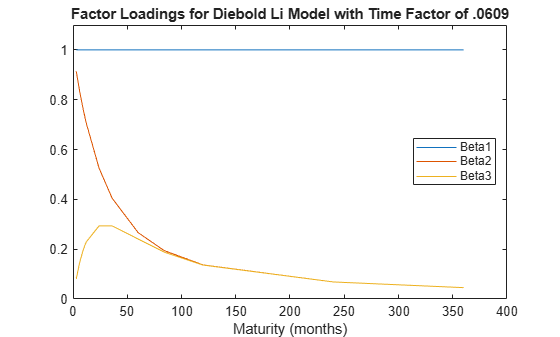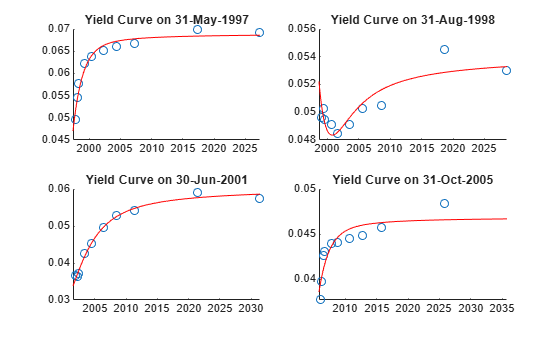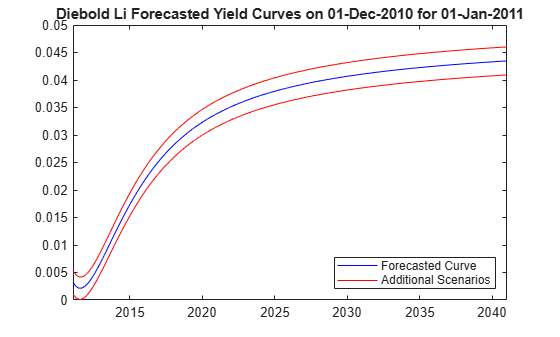Documentation

Fitting the Diebold Li Model

This example shows how to construct a Diebold Li model of the US yield curve for each month from 1990 to 2010. This example also demonstrates how to forecast future yield curves by fitting an autoregressive model to the time series of each parameter.

The paper can be found here:

https://www.nber.org/papers/w10048

The data used are monthly Treasury yields from 1990 through 2010 for tenors of 1 Mo, 3 Mo, 6 Mo, 1 Yr, 2 Yr, 3 Yr, 5 Yr, 7 Yr, 10 Yr, 20 Yr, 30 Yr.

Daily data can be found here:

https://www.treasury.gov/resource-center/data-chart-center/interest-rates/Pages/TextView.aspx?data=yieldAll

Data is stored in a MATLAB® data file as a MATLAB dataset object.

% Extract data for the last day of each month
MonthYearMat = repmat((1990:2010)',1,12)';
EOMDates = lbusdate(MonthYearMat(:),repmat((1:12)',21,1));
MonthlyIndex = find(ismember(Dataset.Properties.ObsNames,datestr(EOMDates)));
Estimationdataset = Dataset(MonthlyIndex,:);
EstimationData = double(Estimationdataset);

Diebold Li Model

Diebold and Li start with the Nelson Siegel model

$y={\beta }_{0}+\left({\beta }_{1}+{\beta }_{2}\right)\frac{\tau }{m}\left(1-{e}^{\frac{-m}{\tau }}\right)-{\beta }_{2}{e}^{\frac{-m}{\tau }}$

and rewrite it to be the following:

${y}_{t}\left(\tau \right)={\beta }_{1t}+{\beta }_{2t}\left(\frac{1-{e}^{-{\lambda }_{t}\tau }}{{\lambda }_{t}\tau }\right)+{\beta }_{3t}\left(\frac{1-{e}^{-{\lambda }_{t}\tau }}{{\lambda }_{t}\tau }-{e}^{-{\lambda }_{t}\tau }\right)$

The above model allows the factors to be interpreted in the following way: Beta1 corresponds to the long term/level of the yield curve, Beta2 corresponds to the short term/slope, and Beta3 corresponds to the medium term/curvature. $\lambda$ determines the maturity at which the loading on the curvature is maximized, and governs the exponential decay rate of the model.

Diebold and Li advocate setting $\lambda$ to maximize the loading on the medium term factor, Beta3, at 30 months. This also transforms the problem from a nonlinear fitting to a simple linear regression.

% Explicitly set the time factor lambda
lambda_t = .0609;

% Construct a matrix of the factor loadings
% Tenors associated with data
TimeToMat = [3 6 9 12 24 36 60 84 120 240 360]';
X = [ones(size(TimeToMat)) (1 - exp(-lambda_t*TimeToMat))./(lambda_t*TimeToMat) ...
((1 - exp(-lambda_t*TimeToMat))./(lambda_t*TimeToMat) - exp(-lambda_t*TimeToMat))];

plot(TimeToMat,X)
title('Factor Loadings for Diebold Li Model with time factor of .0609')
xlabel('Maturity (months)')
ylim([0 1.1])
legend({'Beta1','Beta2','Beta3'},'location','east')Fit the Model

A DieboldLi object is developed to facilitate fitting the model from yield data. The DieboldLi object inherits from the IRCurve object, so the getZeroRates, getDiscountFactors, getParYields, getForwardRates, and toRateSpec methods are all implemented. Additionally, the method fitYieldsFromBetas is implemented to estimate the Beta parameters given a lambda parameter for observed market yields.

The DieboldLi object is used to fit a Diebold Li model for each month from 1990 through 2010.

% Preallocate the Betas
Beta = zeros(size(EstimationData,1),3);

% Loop through and fit each end of month yield curve
for jdx = 1:size(EstimationData,1)
Beta(jdx,:) = [tmpCurveModel.Beta1 tmpCurveModel.Beta2 tmpCurveModel.Beta3];
end

The Diebold Li fits on selected dates are included here

PlotSettles = datenum({'30-May-1997','31-Aug-1998','29-Jun-2001','31-Oct-2005'});
figure
for jdx = 1:length(PlotSettles)
subplot(2,2,jdx)
tmpIdx = find(strcmpi(Estimationdataset.Properties.ObsNames,datestr(PlotSettles(jdx))));
tmpCurveModel = DieboldLi.fitBetasFromYields(PlotSettles(jdx),lambda_t*12,...
hold on
PlottingDates = (PlotSettles(jdx)+30:30:PlotSettles(jdx)+30*360)';
plot(PlottingDates,tmpCurveModel.getParYields(PlottingDates),'r-')
title(['Yield Curve on ' datestr(PlotSettles(jdx))])
datetick
endForecasting

The Diebold Li model can be used to forecast future yield curves. Diebold and Li propose fitting an AR(1) model to the time series of each Beta parameter. This fitted model can then be used to forecast future values of each parameter, and by extension, future yield curves.

For this example the MATLAB function regress is used to estimate the parameters for an AR(1) model for each Beta.

The confidence intervals for the regression fit are also used to generate two additional yield curve forecasts that serve as additional possible scenarios for the yield curve.

The MonthsLag variable can be adjusted to make different period ahead forecasts. For example, changing the value from 1 to 6 would change the forecast from a 1 month ahead to 6 month ahead forecast.

MonthsLag = 1;

[tmpBeta,bint] = regress(Beta(MonthsLag+1:end,1),[ones(size(Beta(MonthsLag+1:end,1))) Beta(1:end-MonthsLag,1)]);
ForecastBeta(1,1) = [1 Beta(end,1)]*tmpBeta;
ForecastBeta_Down(1,1) = [1 Beta(end,1)]*bint(:,1);
ForecastBeta_Up(1,1) = [1 Beta(end,1)]*bint(:,2);
[tmpBeta,bint]  = regress(Beta(MonthsLag+1:end,2),[ones(size(Beta(MonthsLag+1:end,2))) Beta(1:end-MonthsLag,2)]);
ForecastBeta(1,2) = [1 Beta(end,2)]*tmpBeta;
ForecastBeta_Down(1,2) = [1 Beta(end,2)]*bint(:,1);
ForecastBeta_Up(1,2) = [1 Beta(end,2)]*bint(:,2);
[tmpBeta,bint]  = regress(Beta(MonthsLag+1:end,3),[ones(size(Beta(MonthsLag+1:end,3))) Beta(1:end-MonthsLag,3)]);
ForecastBeta(1,3) = [1 Beta(end,3)]*tmpBeta;
ForecastBeta_Down(1,3) = [1 Beta(end,3)]*bint(:,1);
ForecastBeta_Up(1,3) = [1 Beta(end,3)]*bint(:,2);

% Forecasted yield curve
figure
DieboldLi_Forecast = DieboldLi('ParYield',Settle,[ForecastBeta lambda_t*12]);
DieboldLi_Forecast_Up = DieboldLi('ParYield',Settle,[ForecastBeta_Up lambda_t*12]);
DieboldLi_Forecast_Down = DieboldLi('ParYield',Settle,[ForecastBeta_Down lambda_t*12]);
PlottingDates = (Settle+30:30:Settle+30*360)';
plot(PlottingDates,DieboldLi_Forecast.getParYields(PlottingDates),'b-')
hold on
plot(PlottingDates,DieboldLi_Forecast_Up.getParYields(PlottingDates),'r-')
plot(PlottingDates,DieboldLi_Forecast_Down.getParYields(PlottingDates),'r-')
title(['Diebold Li Forecasted Yield Curves on ' datestr(EOMDates(end)) ' for '  datestr(Settle)])
datetickBibliography

This example is based on the following paper:

 Francis X. Diebold, Canlin Li, Forecasting the term structure of government bond yields, Journal of Econometrics, Volume 130, Issue 2, February 2006, Pages 337-364